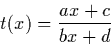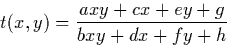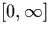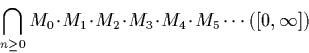Next: Representations Up: Other Possible Representations Previous: B-adic Number Stream

### Linear Fractional Transformations

The linear fractional transformation representation for exact real numbers is described in [10,23,24]. Linear fractional transformations--also known as Möbius transformations--are transformations which are of the following forms:

One dimensional linear fractional transformationos (lft's) are generalisations of linear maps of the form:Two dimensional lft's are transformations of the following form:One useful property of linear fractional transformations is that they can be represented as matrices, and their composition computed using matrix multiplication.

It is possible to use lft's to represent a non negative extended real number as the intersection of an infinite composition of matrices with integer co-efficients applied to the interval.Algorithms for arithmetic operations and transcendental functions can then be developed using this representation.

One advantage with using the linear fractional transformation representation, especially over representations such as the ones using an infinite stream of digits, is that many of the elegant continued fractions for mathematical constants and functions can be used almost directly. It is claimed that this representation is the most efficient for exact real arithmetic, although this claim is as yet unsubstantiated.Next: Representations Up: Other Possible Representations Previous: B-adic Number Stream
Martin Escardo
5/11/2000